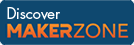Cody

# Problem 250. Project Euler: Problem 10, Sum of Primes

Created by Doug Hull in Community

The sum of the primes below 10 is 2 + 3 + 5 + 7 = 17.

Find the sum of all the primes below the input, N.

Thank you Project Euler Problem 10

### Solution Stats

66.19% Correct | 33.81% Incorrect
Last solution submitted on Mar 15, 2019

#### TagsMATLAB and Simulink resources for Arduino, LEGO, and Raspberry Pi